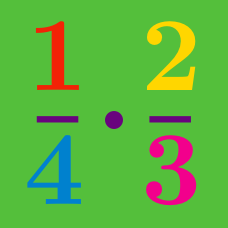Everyday Math

# Fractions - Problem Solving

How many minutes are there in $50\%$ of a third of the entire day?

Details and assumptions

An entire day consists of $24$ hours.

In a certain positive fraction $\frac{a}{b}$, the numerator is 2 less than the denominator. If the numerator and denominator are interchanged, the fraction is increased by $1 \frac{1}{15}$. Find $a + b$.

Details and assumptions

A positive fraction is a fraction that has a positive value. For example, $\frac {10}{12}$ and $\frac { - 2.3}{-0.3}$ are both positive fractions.

It takes $3$ hours for Amy and Beth to water their garden together every weekend. One Saturday, Amy had already been working for $5$ hours alone when Beth woke up late. Then they worked together for $1$ hour(s) to finish the job. If Amy works alone, how many hours would it take to water their garden?

The area of South Korea is $\frac{10}{37}$ of the area of Japan. The area of North Korea is $\frac{12}{25}$ of the area of Texas. If the area of Texas is $\frac{25}{37}$ of that of Japan and the area of Japan is $\frac{37}{982}$ of that of the United States, what fraction of Korean peninsular is South Korea?

Note: All the measurements given in this problem are approximate values.

Find the greatest number given by multiplying three of the following four rational numbers: $-\frac{2}{3}, 21, -4, 18?$

×